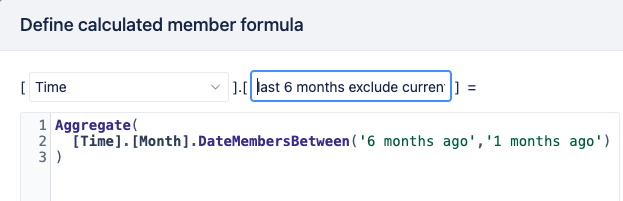# Exclude Current Month from Measure Calculation

I am using the below to calculate 5 months Average Cycle Time. However, this is considering current motnh’s Cycle Time As well. How can I calculate last 6 months Cycle Time excluding current month?

Avg(Filter(
DescendantsSet([Time].CurrentMember,[Time].[Month]),
5) ,
[Measures].[Avg Cycle Time]
)

This might help.
In your Time dimension create a new calculated member as following.
Then use the newly created time member in your measure.1 Like

Thank you @QiZhang . It Worked!